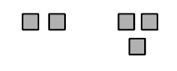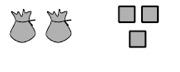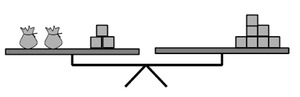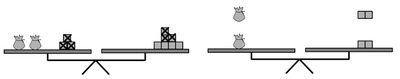# Solving One-Variable Equations

## Objective

Solve equations with variables on both sides of the equal sign.

## Common Core Standards

### Core Standards

?

• 8.EE.C.7 — Solve linear equations in one variable.

?

• 7.EE.B.4

## Criteria for Success

?

1. Understand why solving an equation with variables on both sides of the equal sign follows the same rules as solving an equation with variables on one side.
2. Use inverse operations to solve equations with variables on both sides of the equal sign.
3. Analyze and fix incorrect solutions to equations.

## Tips for Teachers

?

• Solving equations with variables on both sides of the equal sign is new for 8th graders. Though the approach to solving these equations (simplifying, using inverse operations) is the same as what students are used to, there are now more ways that an equation can be solved and additional places where students may have misconceptions (such as combining terms across the equal sign).
• Continue to emphasize the importance of maintaining balance in the equation through each “move” students make.

### Remote Learning Guidance

If you need to adapt or shorten this lesson for remote learning, we suggest prioritizing Anchor Problem 2 (benefits from discussion) and Anchor Problem 3 (benefits from worked example). Find more guidance on adapting our math curriculum for remote learning here.

#### Fishtank Plus

Subscribe to Fishtank Plus to unlock access to additional resources for this lesson, including:

• Problem Set
• Student Handout Editor
• Google Classrom Integration
• Vocabulary Package

## Anchor Problems

?

### Problem 1

In elementary school, students often draw pictures of the arithmetic they do. For instance, they might draw the following picture for the problem ${2+3}$:In this picture, each square represents a tile. We can do the same thing for algebraic expressions, but we need to be careful about how we represent the unknown. If we assume that an unknown number of tiles, $x$, are contained in a bag, we could draw the following picture for $2x+3$:When we have an equation to solve, we assume that the two sides of the equation are equal. We can represent this by showing them level on a balance. For example, the equation $2x+3=7$ could be shown as:When we solve equations, we can add, subtract, multiply or divide both sides of the equation by the same thing in order to maintain the equality. This can be shown in pictures by keeping the balance level. For example, we could solve the equation $2x+3=7$ using pictures by first removing (subtracting) 3 from each side, and then splitting (dividing) the remaining blocks into two equal groups:From this picture, we can see that, in order to keep the balance level, each bag must contain 2 tiles, which means that $x=2$.

1. Solve $5x+1=2x+7$ in two ways: symbolically, the way you usually do with equations, and also with pictures of a balance. Show how each step you take symbolically is shown in the pictures.
2. Solve the equation $4x=x+1$ using pictures and symbols. Discuss any issues that arise.
3. What issues arise when you try to solve the equation $2=2x-4$ using pictures? Do the same issues arise when you solve this equation symbolically?

#### Guiding Questions

Create a free account or sign in to access the Guiding Questions for this Anchor Problem.

#### References

Illustrative Mathematics Solving EquationsParts a-c

Solving Equations, accessed on Aug. 31, 2017, 1:47 p.m., is licensed by Illustrative Mathematics under either the CC BY 4.0 or CC BY-NC-SA 4.0. For further information, contact Illustrative Mathematics.

### Problem 2

The equation below is solved incorrectly three different ways.

For each solution, find and explain the error(s) that occurred. Then find the correct solution for ${ x}$.

${12-4y=7y+10}$

 Incorrect Solution #1 Incorrect Solution #2 Incorrect Solution #3 ${22=3y}$ ${7\frac{1}{3}=y}$ ${4y=7y-2}$ ${-3y=-2}$ ${y=\frac{2}{3}}$ ${4y=7y+22}$ ${-3y=22}$ ${y=-7\frac{1}{3}}$

#### Guiding Questions

Create a free account or sign in to access the Guiding Questions for this Anchor Problem.

### Problem 3

What value for $x$ would make the following equation true?

$20-(3x-9)-2=-(-11x+1)$

#### Guiding Questions

Create a free account or sign in to access the Guiding Questions for this Anchor Problem.

#### References

EngageNY Mathematics Grade 8 Mathematics > Module 4 > Topic A > Lesson 6Example 2

Grade 8 Mathematics > Module 4 > Topic A > Lesson 6 of the New York State Common Core Mathematics Curriculum from EngageNY and Great Minds. © 2015 Great Minds. Licensed by EngageNY of the New York State Education Department under the CC BY-NC-SA 3.0 US license. Accessed Dec. 2, 2016, 5:15 p.m..

## Problem Set

?With Fishtank Plus, you can download a complete problem set and answer key for this lesson. Download Sample

The following resources include problems and activities aligned to the objective of the lesson that can be used to create your own problem set.

## Target Task

?

Three expressions are shown below.

 Expression A: $-\left(8-\frac{1}{2}x\right)$ Expression B: $3-1.5x$ Expression C: $-2+6x-6$

Using the expressions in the chart, find the value of $x$ in each situation described.

a.   A = C

b.   A + B = C

#### Mastery Response

Create a free account or sign in to view Mastery Response